A Worked Example: Common Tangent Construction and Spinodal Points for a Single Curve
(Part A: The Spinodal Region)

A prototype molar free energy of mixing using the same xlogx function for the ideal entropy of mixing terms.  The temperature term is a scaled energy (RT), and it is assumed that enthalpies have been scaled so that the temperatures of interest (if there are any) are between T=0 and T=10.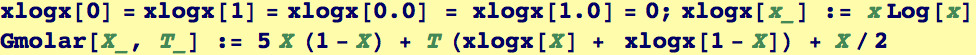Here is the shape of our prototype free energy at T=3/2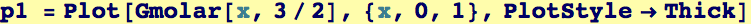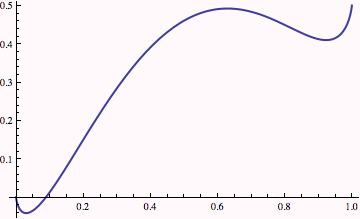We will need the bounds of the above graphics object: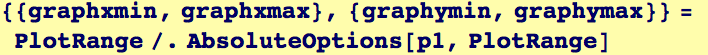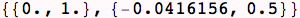First let's determine where the spinodal region (by finding where the second derivative with respect to composition is negative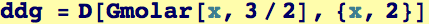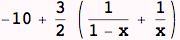Then, use RegionPlot to illustrate the range over which spinodal decomposition is spontaneous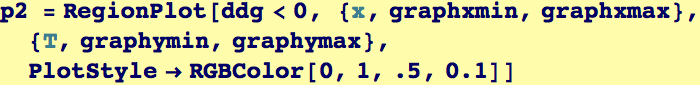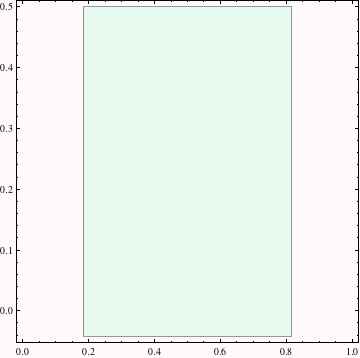Show them both together to identify the spinodal region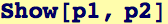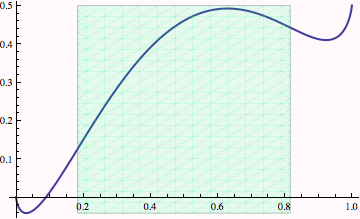Created by Wolfram Mathematica 6.0  (28 August 2007)